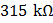# Following figure shows cross-sections through three long conductors of the same length and material, with square cross-section of edge lengths as shown. Conductorwill fit snugly within conductorand conductorwill fit snugly within conductorRelationship between their end to end resistance isa)b)c)d) Information is not sufficient

## Question ID - 150323 :- Following figure shows cross-sections through three long conductors of the same length and material, with square cross-section of edge lengths as shown. Conductorwill fit snugly within conductorand conductorwill fit snugly within conductorRelationship between their end to end resistance isa)b)c)d) Information is not sufficient

3537

 (a) All the conductors have equal lengths. Area of cross-section ofisSimilarly area of cross-section ofArea of cross-section ofHence according to formularesistances of all the conductors are equalAvoltmeter of internal resistancein series with a high resistanceis connected to aline. The voltmeter readsthe value ofis a)b)c)d)